# Program to check Sphenic Number in Java

In this tutorial you will learn about the Program to check Sphenic Number in Java and its application with practical example.

In this tutorial, we will learn to create a Java Program to check Sphenic Numbers in Java using Java programming.

## Prerequisites

Before starting with this tutorial we assume that you are best aware of the following Java programming topics:

• Java Operators.
• Basic Input and Output function in Java.
• Class and Object in Java.
• Basic Java programming.
• If-else statements in Java.
• For loop in Java.

## What is Sphenic Number ?

Sphenic numbers are numbers which have exactly 3 factors,and all are prime number.
Example :Some of the Sphenic Number are

Number => 30: has Factor’s 2,3,5 and product of 2*3*5= 30 So it is a Sphenic number.
and             42: has Factor’s 2,3,7 and product of 2*3*7= 42 So it is not  a Sphenic number.
15: has Factor’s 3,5 and product of 3*5= 15  So it is not a Sphenic number.
Number > 70: has Factor’s 1,7,10 and product of 1*7*10= 70  So it is a Sphenic number.

## Program to check Sphenic Number in Java.

In this program we will find given number is a Sphenic number or not in using java programming. We would first declared and initialized the required variables. Next, we would prompt user to input  a number.Later we  find number is Sphenic number or not let’s have a look at the code.

## Output

Sphenic Number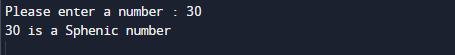Not a Sphenic Number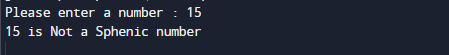In the above program, we have first declared and initialized a set variables required in the program.

• number= it will hold entered number.

After declaring variables in the next statement user will be prompted to enter a value and  which will be assigned to variable ‘number’.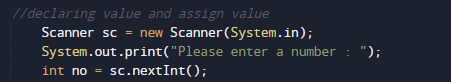So, first of all we will try to find the number is having  three digits (except 1) are prime or not.and product of these number gives the number or not .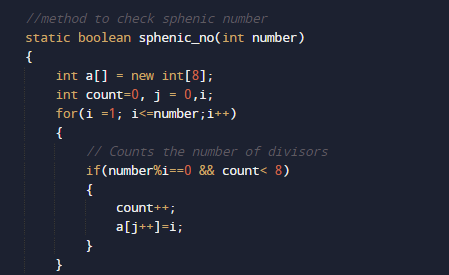we can say that  number n is a sphenic if  n=a x b x c (a,b, and c are three distinct prime numbers and their product are n)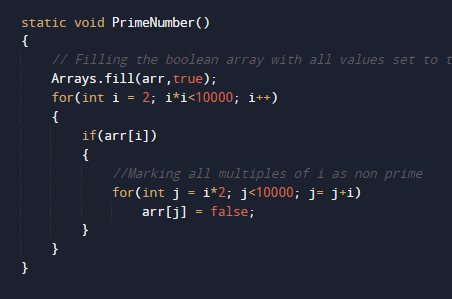After all these condition we can say a number follow all this is a Sphenic number.

In this tutorial we have learn about the Program to check Sphenic Number in Java and its application with practical example. I hope you will like this tutorial.